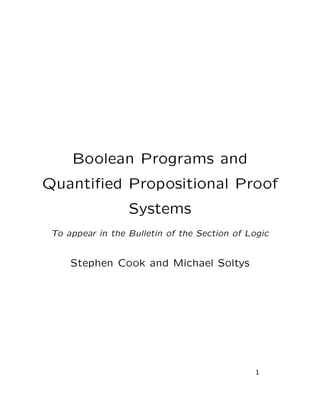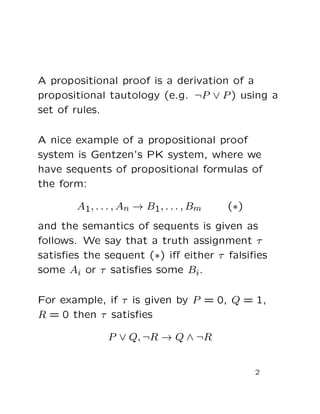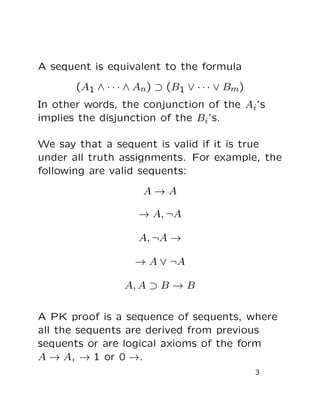Successfully reported this slideshow.

# Boolean Programs and Quantified Propositional Proof System -×

1 of 17
1 of 17

# Boolean Programs and Quantified Propositional Proof System -

## More Related Content

### Boolean Programs and Quantified Propositional Proof System -

1. 1. Boolean Programs and Quantiﬁed Propositional Proof Systems To appear in the Bulletin of the Section of Logic Stephen Cook and Michael Soltys 1
2. 2. A propositional proof is a derivation of a propositional tautology (e.g. ¬P ∨ P) using a set of rules. A nice example of a propositional proof system is Gentzen’s PK system, where we have sequents of propositional formulas of the form: A1, . . . , An → B1, . . . , Bm (∗) and the semantics of sequents is given as follows. We say that a truth assignment τ satisﬁes the sequent (∗) iﬀ either τ falsiﬁes some Ai or τ satisﬁes some Bi. For example, if τ is given by P = 0, Q = 1, R = 0 then τ satisﬁes P ∨ Q, ¬R → Q ∧ ¬R 2
3. 3. A sequent is equivalent to the formula (A1 ∧ · · · ∧ An) ⊃ (B1 ∨ · · · ∨ Bm) In other words, the conjunction of the Ai’s implies the disjunction of the Bi’s. We say that a sequent is valid if it is true under all truth assignments. For example, the following are valid sequents: A → A → A, ¬A A, ¬A → → A ∨ ¬A A, A ⊃ B → B A PK proof is a sequence of sequents, where all the sequents are derived from previous sequents or are logical axioms of the form A → A, → 1 or 0 →. 3
4. 4. PK Rules of inference: weak Γ → ∆ A, Γ → ∆ Γ → ∆ Γ → ∆, A exch Γ1, A, B, Γ2 → ∆ Γ1, B, A, Γ2 → ∆ Γ → ∆1, A, B, ∆2 Γ → ∆1, B, A, ∆2 cont Γ, A, A → ∆ Γ, A → ∆ Γ → ∆, A, A Γ → ∆, A “¬” Γ → ∆, A ¬A, Γ → ∆ A, Γ → ∆ Γ → ∆, ¬A “∧” A, B, Γ → ∆ (A ∧ B), Γ → ∆ Γ → ∆, A Γ → ∆, B Γ → ∆, (A ∧ B) “∨” A, Γ → ∆ B, Γ → ∆ (A ∨ B), Γ → ∆ Γ → ∆, A, B Γ → ∆, (A ∨ B) cut Γ → ∆, A A, Γ → ∆ Γ → ∆ 4
5. 5. Any valid sequent can be derived from initial sequents of the form A → A, → 1 and 0 →, using the above rules (completeness of PK), and only valid sequents can be derived (soundness of PK). As an example we give a proof of one of DeMorgan’s laws: ¬(P ∧ Q) → ¬P ∨ ¬Q Here is the derivation: P → P → P, ¬P → P, ¬P, ¬Q Q → Q Q → Q, ¬P → Q, ¬P, ¬Q → P ∧ Q, ¬P, ¬Q → P ∧ Q, ¬P ∨ ¬Q ¬(P ∧ Q) → ¬P ∨ ¬Q 5
6. 6. Abstract Deﬁnition: A proof system is a feasible (i.e. easily computable) map f : {0, 1}∗ onto −→ {tautologies} Where {tautologies} is just the set of propositional tautologies, and if f(x) = A then x is the proof of the propositional tautology A. A proof system is polynomially bounded if there exists a polynomial p such that for every A ∈ {tautologies}, there exists an x such that |x| ≤ p(|A|), i.e. every tautology has a proof which is “proportional” to its size. See “The Complexity of Propositional Proofs”, by Alasdair Urquhart, in The Bulletin of Symbolic Logic, December 1995, for all the details. 6
7. 7. Interest in propositional proof complexity arose from two ﬁelds connected with computers: automated theorem proving (artiﬁcial intelligence) and computational complexity theory. Some Complexity Theory P is the class of problems that can be solved with algorithms that run in polynomial time in the size of the input (e.g. given a truth assignment τ, and a sequent S, does τ satisfy S?). NP is the class of problems whose solutions can be veriﬁed by an algorithm that runs in polynomial time (e.g. given a sequent S, is it satisﬁable?) co-NP is the class of problems for which a counter-example can be veriﬁed by an algorithm that runs in polynomial time (e.g. given a sequent S, is it valid?). 7
8. 8. Theorem: NP = co-NP iﬀ there is a polynomially bounded proof system for the propositional tautologies. Also P = NP implies NP = co-NP, thus if we prove that there is no polynomially bounded proof system (which is what experts conjecture), then it will follow that NP = co-NP, and therefore P = NP. The problem P ? = NP has been listed by Steve Smale in the top three “Mathematical Problems for the Next Century” (Math Intelligencer 20, 1998). 8
9. 9. How could we prove that there is no polynomially bounded proof system? It seems like a very diﬃcult problem. The standard approach is to consider stronger and stronger proof systems, and to exhibit superpolynomial lower bounds for each of them. strongest Quantiﬁed PK Extended PK PK ———————— Bounded Depth PK Resolution weakest Truth Tables However, we can only prove superpolynomial lower bounds for the weaker systems (the ones below the line). The systems above the line have no known lower bounds. 9
10. 10. Quantiﬁed PK Quantiﬁed propositional PK is formed by introducing propositional quantiﬁers: ∀xA(x) whose meaning is A(0) ∧ A(1) ∃xA(x) whose meaning is A(0) ∨ A(1) The propositional quantiﬁers do not increase the expressive power of formulas. Instead, they allow us to shorten some propositional formulas. For example, the formula (x1,...,xn)∈{0,1}n A(x1, . . . , xn) has size in the order of 2n|A|, but the equivalent quantiﬁed formula ∃x1 . . . ∃xnA(x1, . . . , xn) has size in the order of n + |A|. 10
11. 11. The quantiﬁed propositional calculus is ﬁrst discussed by B. Russell in “The Theory of Implication”, American Journal of Mathematics in 1906. Also, the Polish mathematician Le´sniewski treated it in his “Protothetic”. Martin Dowd investigated the connection between quantiﬁed propositional proof systems and the complexity class PSPACE in his PhD thesis in 1979. A modest source of information is also a 5 page section in Jan Kraj´ıˇcek’s book “Bounded Arithmetic, Propositional Logic, and Complexity Theory”, 1995. Still, little is known about quantiﬁed propositional proof systems. 11
12. 12. G is PK with ∃ and ∀ I follow the results and notation from Jan Kraj´ıˇcek’s “Bounded Arithmetic, Propositional Logic, and Complexity Theory”. The quantiﬁed propositional proof system G extends LK by allowing quantiﬁed propositional formulas in sequents, and by adding the following quantiﬁer rules: A(B), Γ → ∆ ∀xA(x), Γ → ∆ Γ → ∆, A(p) Γ → ∆, ∀xA(x) A(p), Γ → ∆ ∃xA(x), Γ → ∆ Γ → ∆, A(B) Γ → ∆, ∃xA(x) where B is any formula, and with the restriction that the atom p does not occur in the lower sequents of ∀-right and ∃-left. 12
13. 13. Let G1 be the restriction of G where we allow only strict Σ q 1 formulas, that is, all formulas must begin with a block of existential quantiﬁers (possibly empty) followed by a quantiﬁer free formula. In other words, all formulas are either quantiﬁer free, or of the form ∃x1 . . . ∃xnA(x1, . . . , xn) where A(x1, . . . , xn) is quantiﬁer free. For example, the sequent ∃x∃y((¬x ∨ p) ∧ (¬y ∨ q)) → ∃x(x ∨ ¬p ∨ q), p ∧ q has only Σ q 1 formulas. 13
14. 14. Witnessing Given a sequent S, we want to have a way of computing the values of the existential variables on the right in terms of the free variables, and the quantiﬁed variables on the left. For example, consider the sequent ∃yA(x, y) → ∃yA(x, y) (∗) where x, y are the only variables in this sequent. In this case it is very easy. The quantiﬁed variable y on the right can be witnessed by the following function: f(x, y) = y that is, the sequent A(x, y) → A(x, f(x, y)) is valid if (∗) was valid. 14
15. 15. Suppose that π is a G1 proof. We show that the existential quantiﬁers can be witnessed by Boolean programs. We do this by induction on the number of sequents in π. For example, consider the contraction rule: C(¯p) → D(¯p), ∃xB(x), ∃xB(x) C(¯p) → D(¯p), ∃xB(x) By Induction Hypothesis, we know that there are Boolean programs g, h such that the sequent C(¯p) → D(¯p), B(g(¯p)), B(h(¯p)) is valid. Now, deﬁne f as follows: f(¯p) =    g(¯p) if B(g(¯p)) h(¯p) otherwise The bottom sequent can thus be converted to the valid sequent C(¯p) → D(¯p), B(f(¯p)), 15
16. 16. Main Idea If π is a G1 proof of a propositional tautology, and π is tree-like, then the existential quantiﬁers can be feasibly computed by evaluating Boolean circuits. This was already shown in Jan Kraj´ıˇcek’s book “Bounded Arithmetic, Propositional Logic, and Complexity Theory”. If, on the other hand, π is not tree-like, then it seems that evaluating the existential quantiﬁers requires a lot of computational power, i.e. Boolean programs whose evaluation is a PSPACE complete problem. This is shown in our paper “Boolean Programs and Quantiﬁed Propositional Proof Systems”. 16
17. 17. References “Boolean Programs and Quantiﬁed Propositional Proof Systems”, by S.Cook and M. Soltys, in this paper we introduce the notion of Boolean programs, and we characterize PSPACE in terms of families of Boolean programs. We then show how to use Boolean programs to witness quantiﬁers in G1. “The Complexity of Propositional Proofs”, by Alasdair Urquhart, in The Bulletin of Symbolic Logic, December 1995, a comprehensive introduction to the complexity of propositional proofs. “Bounded Arithmetic, Propositional Logic, and Complexity Theory”, by Jan Kraj´ıˇcek. A short introduction to quantiﬁed propositional proof systems. 17